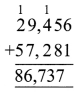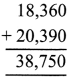# DAV Class 4 Maths Chapter 2 Worksheet 4 Solutions

The DAV Class 4 Maths Book Solutions and DAV Class 4 Maths Chapter 2 Worksheet 4 Solutions of Addition and Subtraction offer comprehensive answers to textbook questions.

## DAV Class 4 Maths Ch 2 WS 4 Solutions

Question 1.
Solve the following word problems.
(a) In an examination, 29,456 candidates passed while 57,281 candidates failed. How many candidates appeared for the examination?
Number of candidates passed = 29,456
Number of candidates failed = 57,281
Total candidates appeared = 86,737Thus, 86,737 candidates appeared for the examination.

(b) In a school library, there are 11,250 Science books, 21,312 Maths books and 20,143 Hindi books. Find the total number of books in the library.
Number of Science books = 11,250
Number of Maths books = 21,312
Number of Hindi books = 20,143
Total number of books = 52,705Thus, total number of books in the library is 52,705.(c) In a school, there are 1,752 students in primary classes, 1,825 in middle classes and 1,790 in senior classes. Find the total strength of school.
No. of students in primary classes = 1,752
No. of students in middle classes = 1,825
No. of students in senior classes = 1,790
Total strength = 5,367Thus, total strength of school is 5,367.

(d) The cost of a black and white T.V. set is ₹ 3,690. The cost of a colour T.V. set is ₹ 12,810 more than the cost of black and white T.V. set. Find the total cost of both the T.V. sets.
Cost of black and white T.V. set = ₹ 3,690
Cost of colour T.V. is ₹ 12,810 more than black and white T.V.
So, cost of colour T.V. = ₹ 23,690 + ₹ 12,810
= ₹ 16,500
Total cost of both T.V. sets
= ₹ (16,500 + 3,690)
= ₹ 20,190
Thus, cost of both the T.V. sets are ₹ 20,190.

(e) Raman purchased a house for ₹ 6,65,700. He spent ₹ 2,975 on its repairs. Find the amount he spent in all.
Cost of house = ₹ 6,65,700
Amount spent on repairing = ₹ 2,975
Total amount spent = ₹ 6,68,675
Thus, the amount he spent in all is ₹ 6,68,675.

### DAV Class 4 Maths Chapter 2 Worksheet 4 Notes

Word Problems

In our daily life we use addition in many situations.
E.g.1:
A factory manufactured 18,360 nails in 1st week of March and 20,390 nails in 2nd week of March. Find out the total nails manufactured in two weeks.
Nails manufactured in 1st week = 18,360
Nails manufactured in 2nd week = 20,390
Total nails manufactured = 38,750E.g. 2:
An election was contested by Rohan and Jacob Rohan got 86,700 votes and Jacob got 90,300 votes. Find the total votes received by Jacob and Rohan.